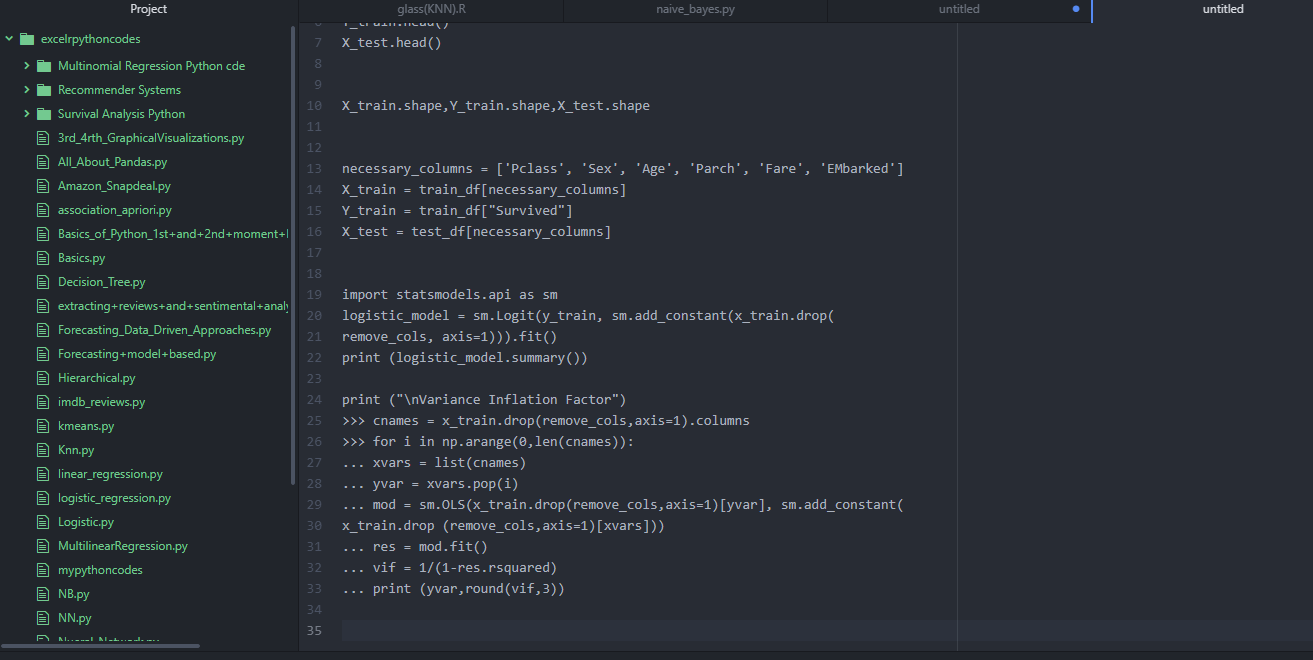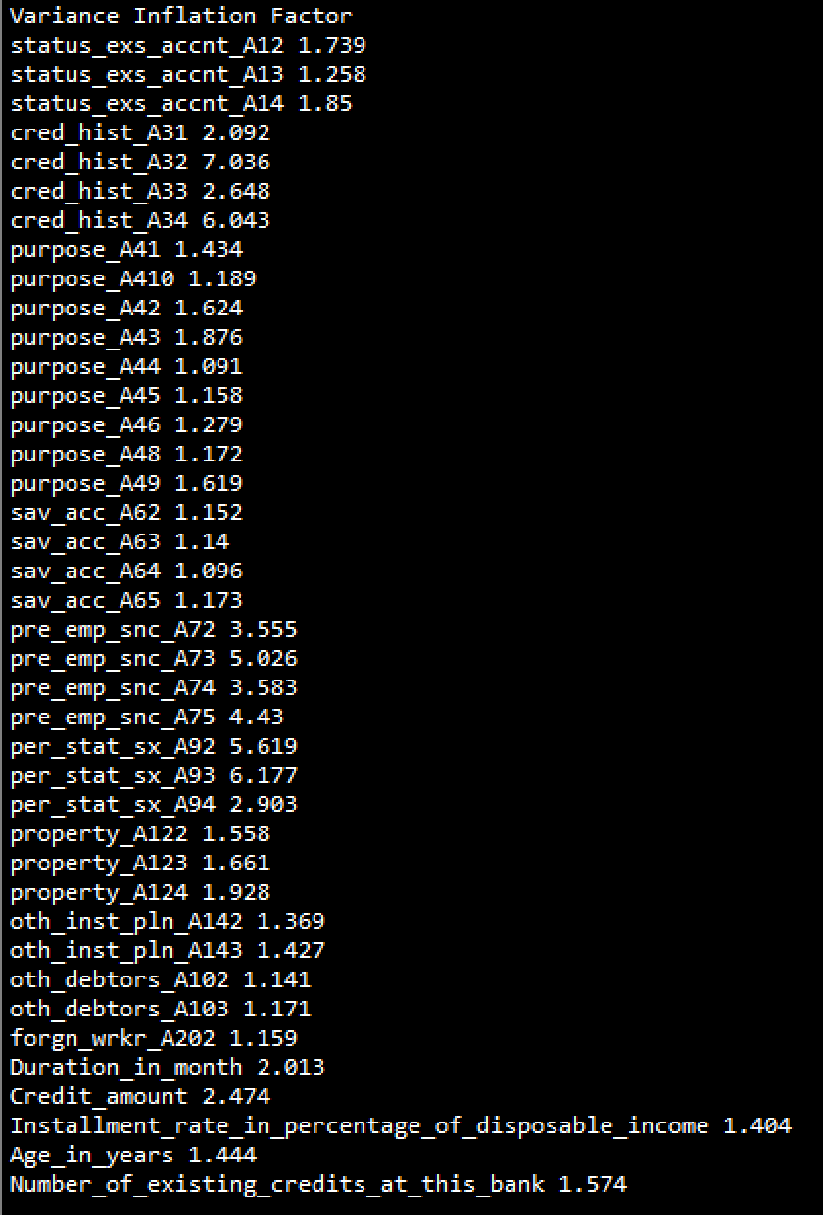# What are the parameters considered for checking multicollinearity issues?

596    Asked by shivangiMehta in Data Science , Asked on Nov 30, 2019

The below parameters are considered for multicollinearity issues.

p-value- This parameter can give us an idea which variables are highly co linear. A higher p-value indicates that a variable is the most insignificant among other variables and do not cause much effect to the model if we remove it.

VIF factor- VIF values are calculated to check multicollinearity, although insignificant variables need to be removed prior to the removal of multicollinear variables with VIF > 5.

The following code gives a glimpse of finding out VIF factorThe output is as followsFrom the following results, Per_stat_sx_A93 is coming in as the most multi-collinear variable with a VIF of 6.177. So in order to remove multicollinearity issues, we can remove it.